Refer to our Texas Go Math Grade 3 Answer Key Pdf to score good marks in the exams. Test yourself by practicing the problems from Texas Go Math Grade 3 Module 18 Assessment Answer Key.

Vocabulary

Choose the best term from the box.

Vocabulary
capacity
kilograms
liquid volume
liters

Question 1.
_________ is the amount a container can hold. (p, 589)
Capacity

Explanation:
Capacity is the amount a container can hold.

Question 2.
Mass can be measured in _________. (p. 607)
Kilograms.

Explanation:
Mass can be measured in kilograms.

Concepts and Skills

Locate and draw points at $$\frac{1}{4}$$, $$\frac{3}{4}$$, and $$\frac{2}{2}$$. Write the fraction that Is closest to zero and farthest from zero. (TEKS 3.3.B, 3.7.A)
Question 3.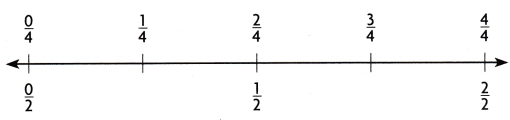closest _____________
farthest ____________
Closest $$\frac{1}{4}$$
farthest $$\frac{2}{2}$$ or $$\frac{4}{4}$$

Explanation: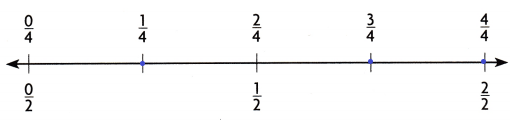Choose the best unit to measure each capacity. Write cup, pint, quart, or gallon. (TEKS 3.7.D, 3.7.E)

Question 4.bathtub __________
Gallon

Explanation:
The best unit to measure each capacity is Gallon.

Question 5.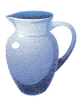pitcher ____________
Quart

Explanation:
The best unit to measure each capacity is Quart.

Question 6.a dog bowl _________
Cup

Explanation:
The best unit to measure each capacity is Cup.

Choose the unit you would use to measure the mass. Write gram or kilogram. (TEKS 3.7.D, 3.7.E)

Question 7.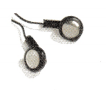earphones ___________
Gram

Explanation:
The best unit to measure each capacity is Gram.

Question 8.lamp ___________
Kilogram

Explanation:
The best unit to measure each capacity is Kilogram.

Question 9.boots ___________
Kilogram

Explanation:
The best unit to measure each capacity is Kilogram.

Fill in the bubble for the correct answer choice.

Question 10.
At soccer practice, Valerie ran for 13 minutes and practiced drills for 20 minutes. She left practice at 2:15 P.M. At what time did Valerie arrive at soccer practice? (TEKS 3.7.C)
(A) 1:33 P.M.
(B) 1:42 P.M.
(C) 2:03 P.M.
(D) 2:48 P.M.
1:42 P.M

Explanation:
Valerie ran for 13 minutes and practiced drills for 20 minutes the total time is 13+20 = 33 minutes. And she left for practice at 2:15 P.M. Hence Valerie arrive at soccer practice at 1:42 P.M.

Question 11.
Dora placed a pencil on one side of a balance. Which is the mass of the pencil? (TEKS 3.7.D, 3.7.E)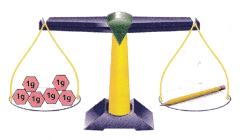(A) 1 gram
(B) 6 grams
(C) 1 kilogram
(D) 6 kilograms
6 grams

Explanation:
The mass of the pencil is 6 grams.

Question 12.
Arielle and Vienna baked a pan of brownies. Then they divided the pan of brownies into 8 equal squares, each weighing 2 ounces. Which is the combined weight of the 8 brownies? (TEKS 3.7.D, 3.7.E)
(A) 1 pound
(B) 2 ounces
(C) 8 ounces
(D) 2 pounds
1 pound

Explanation:
The combined weight of the 8 brownies is 1 pound.

Question 13.
Which measurement unit would you use to find the total liquid volume of this container? (TEKS 3.7.D, 3.7.E)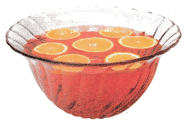(A) pounds
(B) milliliter
(C) liters
(D) grams
Liters

Explanation:
The measurement unit would you use to find the total liquid volume of this container is in liters.

Question 14.
Allen started his homework at 8:10 P.M. and worked for 45 minutes. Then he phoned a friend and talked for 20 minutes. Allen went to bed at 9:15 P.M. How much time elapsed from Allen starting his homework till he went to bed? (TEKS 3.7.C)
(A) 15 minutes
(B) 45 minutes
(C) 65 minutes
(D) 20 minutes